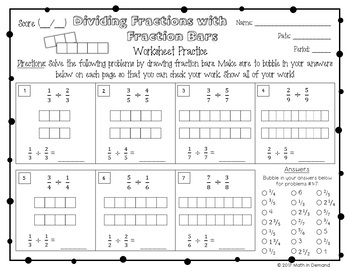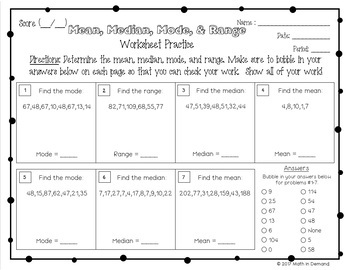# 6th Grade Math Practice Worksheets (Entire Year)

Rated 4.86 out of 5, based on 22 reviews
22 Ratings;
6th
Subjects
Resource Type
Formats Included
• Zip
Pages
\$39.99
\$39.99
Report this resource to TPT

#### What educators are saying

I enjoyed the other products so much that I just had to get these too. As usual, they did not disappoint! These pages were great for extra practice/review!
I needed a daily review sheet for a few of my higher grade level students that was set up the same way each day due to the disability. This was perfect and simple set up was key! Thank you!
##### Also included in
1. ⭐ This bundle includes ALL of my 6th Grade Math material in my store and ALL future 6th Grade Math Products ⭐This includes all of my popular 6th grade math interactive notebooks, practice worksheets, foldables, activities, warm-ups, homework, early finishers, exit slips, assessments, and SO MUCH MOR
Price \$249.99Original Price \$388.40Save \$138.41
2. ⭐ This bundle includes ALL of my Math Curriculum in my Teachers Pay Teachers store ⭐You will be receiving ALL of my 6th, 7th, and 8th Grade Math interactive notebooks, activities, foldables, practice worksheets, warm-ups, exit slips, assessments, and much more! This is well over 1000 pages of materi
Price \$899.99Original Price \$1718.65Save \$818.66
3. This listing is for a whole years worth of practice worksheets for 6th, 7th, and 8th grade math (some worksheets are still in progress). That is 163 math worksheets when all worksheets are completed!!! These worksheets are self assessing because they include a bubble-in area for the answer key. Som
Price \$74.99Original Price \$119.97Save \$44.98

### Description

This is a whole years worth of practice worksheets for 6th grade math. These worksheets are self assessing because they include a bubble-in area for the answer key. There are over 45 worksheets! The worksheets are broken up into 5 units that are perfectly aligned to my 6th grade math interactive notebook. These 5 units include:

Unit 1 - Number Sense

Unit 2 – Ratios, Rates, and Percentages

Unit 3 - Expressions & Equations

Unit 4 - Geometry

Unit 5 - Statistics & Probability

A breakdown of each unit is below:

Unit 1 - Number Sense

-Subtracting Decimals

-Multiplying Decimals

-Dividing Decimals

-Dividing Fractions with Fraction Bars

-Dividing Fractions

-Word Problems involving Dividing Fractions

-Exponents

-Order of Operations

-Intro to Negative Numbers & Comparing Negative Numbers

-Positive and Negative Numbers

-Number Opposites

-Absolute Value

-Coordinate Plane

-Distance between Points with a Same Coordinate

-Least Common Multiple & Greatest Common Factor

Unit 2 - Ratios, Rates, & Percentages

-Intro to Ratios & Word Problems

-Intro to Rates & Word Problems

-Intro to Percents

-Percent-Decimal Conversions

-Percent of a Number - Finding an Amount

-Finding a Percent

-Finding the Base

Unit 3 - Expressions & Equations

-Intro to Variables

-Evaluating Expressions

-Writing Algebraic Expressions

-Solving One-Step Equations

-Intro to Inequalities

-Solving One-Step Inequalities

-Dependent vs Independent Variables

-Combining Like Terms

-The Distributive Property

-Writing Equivalent Expressions

Unit 4 - Geometry

-Area of Parallelograms

-Area of Triangles

-Area of Trapezoids

-Area of Composite Figures

-Identifying Parts of 3-Dimensional Objects

-Volume of Rectangular Prisms (Fractional Lengths)

-Surface Area using Nets

-Polygons in the Coordinate Plane

Unit 5 - Statistics & Probability

-Dot Plots & Frequency Tables

-Histograms

-Mean, Median, Mode, and Range

-Box Plots (includes IQR)

Total Pages